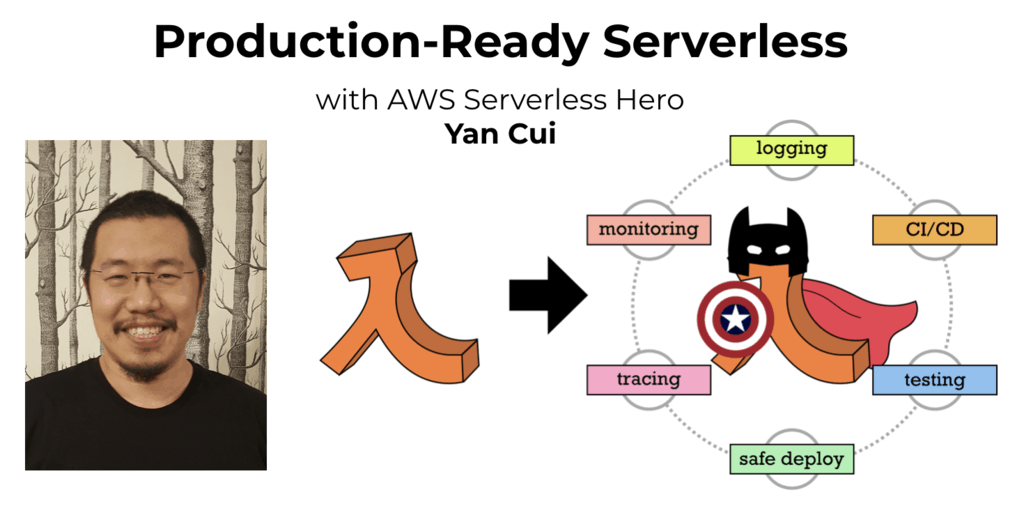# Project Euler – Problem 33 Solution

Check out my new course Learn you some Lambda best practice for great good! and learn the best practices for performance, cost, security, resilience, observability and scalability.

#### Problem

The fraction 49/98 is a curious fraction, as an inexperienced mathematician in attempting to simplify it may incorrectly believe that 49/98 = 4/8, which is correct, is obtained by cancelling the 9s.

We shall consider fractions like, 30/50 = 3/5, to be trivial examples.

There are exactly four non-trivial examples of this type of fraction, less than one in value, and containing two digits in the numerator and denominator.

If the product of these four fractions is given in its lowest common terms, find the value of the denominator.

#### Solution

```// define function to check if two numbers has the cancelling behaviour
let isCancelling a b =
let aStr, bStr = a.ToString(), b.ToString()
if aStr. = bStr. then double(aStr..ToString())/double(bStr..ToString()) = double(a)/double(b)
else if aStr. = bStr. then double(aStr..ToString())/double(bStr..ToString()) = double(a)/double(b)
else if aStr. = bStr. then double(aStr..ToString())/double(bStr..ToString()) = double(a)/double(b)
else if aStr. = bStr. then double(aStr..ToString())/double(bStr..ToString()) = double(a)/double(b)
else false

// define function to find the greatest common dividor
let gcd a b = [2..min a b] |> List.rev |> Seq.filter (fun x -> a % x = 0 && b % x = 0) |> Seq.head

// define function that returns numbers >= n which are not multiples of 10
// and have two distinct digits (i.e. 11, 22 only have one distinct digits)
let numbers n = [n..99] |> List.filter (fun x -> x % 10 <> 0 && (x % 10 <> x/10))

// first work out the product of all 4 fractions, in fractions form (num, denom)
let fraction =
numbers 10
|> List.collect (fun x -> numbers (x+1) |> List.map (fun y -> (x, y)))
|> List.filter (fun (x, y) -> isCancelling x y)
|> List.reduce (fun (num, denom) (x, y) -> (num*x, denom*y))
// then define the denominator by the greatest common divisor
(snd fraction) / gcd (fst fraction) (snd fraction)
```Hi, I’m Yan. I’m an AWS Serverless Hero and the author of Production-Ready Serverless.

I specialise in rapidly transitioning teams to serverless and building production-ready services on AWS.

Are you struggling with serverless or need guidance on best practices? Do you want someone to review your architecture and help you avoid costly mistakes down the line? Whatever the case, I’m here to help.Check out my new course, Learn you some Lambda best practice for great good! In this course, you will learn best practices for working with AWS Lambda in terms of performance, cost, security, scalability, resilience and observability. Enrol now and enjoy a special preorder price of £9.99 (~\$13).Are you working with Serverless and looking for expert training to level-up your skills? Or are you looking for a solid foundation to start from? Look no further, register for my Production-Ready Serverless workshop to learn how to build production-grade Serverless applications!